## Equilibrium Concentrations

In a system that has reached chemical equilibrium, the concentrations of the various substances are quantitatively related. In the example of transformations between the two nitrogen oxides, the concentrations at equilibrium obey this equilibrium expression: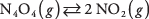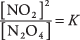where the value K is the equilibrium constant.

For any equilibrium reaction, the ratio of concentrations of the substances on the right to the concentrations of those on the left equals a constant appropriate for that specific reaction. Notice that the ratio is always written with the products over the reactants. Each concentration must be raised to the power of its stoichiometric coefficient in the reaction. Because NO 2 has a coefficient of 2 in the reaction, its concentration must be squared in the preceding expression.

For a generalized reaction written

w A + x B → y C + z D

where the lowercase letters represent numerical coefficients for the balanced reaction, the equilibrium constant is calculated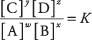where the brackets denote concentrations of the various substances. Therefore, the reaction of nitrogen and hydrogen to yield ammoniaobeys this equilibrium equation:The dissociation of water vapor to hydrogen and oxygenfollows this equation: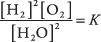Of course, the equilibrium constant K in the latter equation does not have the same value as the K in the equilibrium equation for ammonia. The numerical value of K depends on the particular reaction, the temperature, and the units used to describe concentration. For liquid solutions, the concentrations are usually expressed as molarity. For a mixture of gases, the concentration of each molecular species is commonly given either as molarity or as pressure in atmospheres.

Use the ammonia equilibrium,where the concentrations are measured as pressures. At 25°C, one study reported the equilibrium pressures of the three gases. See Table 1.From the experimental data in Table 1, you can calculate the equilibrium constant for the reaction at 25°C.Equilibrium constants have been determined for many reactions over a wide range of temperatures. One obvious use is to calculate the concentrations of the various substances at equilibrium. In the ammonia example, if you had been given the equilibrium constant K and the pressures of ammonia and hydrogen, you could have calculated the pressure of nitrogen. (You may want to attempt this simple calculation.)

Another important use of equilibrium constants is to predict the initial direction of a reaction. Most commonly, the original concentrations of substances are unstable with respect to equilibrium; this is necessarily the case if any substance is absent. The reaction will proceed in one direction until equilibrium is attained. Referring to the ammonia example, if initially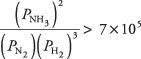ammonia decomposes to nitrogen and hydrogen with decreasing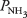and increasinganduntil the equilibrium pressures are reached. In the converse case, where initiallynitrogen and hydrogen will combine to form ammonia with decreasing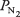andand increasinguntil the values satisfy the equilibrium constant. Notice that only concentrations at equilibrium are stable and unchanging at a given temperature.

Although equilibrium calculations involve the concentrations of dissolved substances and gases, the values for pure liquids and solids are virtually constant and so are usually not incorporated into the equilibrium constant. As an illustration of this point, the reaction \contains a liquid (H 2O) that has a fixed composition. The equilibrium may be expressed by either of the two equations: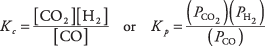where the concentration of H 2O does not appear because, as a constant, it is included in the value of the equilibrium constant. As was mentioned earlier, there are different equilibrium constants depending on whether the concentrations are in molarity ( K c) or pressure ( K p).

As one more example of the nonappearance of pure phases in the equilibrium equation, examine the reaction of lithium bromide with hydrogen:The equilibrium equation isConstant concentrations of the two solids (lithium bromide and lithium hydride) are not included in the value of K. Although pure liquids and solids do not appear in the equilibrium expression as commonly written, they must be present as real substances in the actual reaction for the two opposing reactions to be at equilibrium.

The earlier calculations for both acid dissociations and solubility products are special applications of finding concentrations from equilibrium constants.

• At 100°C, the halogen reactionhas an equilibrium constant of 0.15 when the concentrations are expressed in either molarity or atmospheres. A 1.0 liter reaction vessel is filled with 100 grams of Br 2 and 50 grams each of Cl 2 and BrCl. Do an equilibrium calculation to predict the direction of the initial reaction.

• At 700°C, the reactionhas an equilibrium constant K p = 3.76 when the concentrations of the gases are expressed in atmospheres. Ifand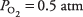what is the pressure of SO 3? Assume that the gases are at equilibrium.